Question

There are two categories of ultraviolet light. Ultraviolet A (UVA) has a wavelength ranging from 320 nm to 400 nm. It is necessary for the production of vitamin D. UVB, with a wavelength in vacuum between 280 nm and 320 nm, is more dangerous because it is much more likely to cause skin cancer.

(a) find the frequency ranges of UVA and UVB.

(b) what are the ranges of the wave numbers for UVA and UVB?

1.ngockhue

(a) UVA – frequency range: 7.5*10^14 Hz to 9.37*10^14 Hz

UVB – frequency range: 9.37*10^14Hz to 1.07*10^15Hz

(b) UVA – wavenumber range: 1.57*10^7 m^-1 to 1.96*10^7m^-1

UVB – wavenumber range: 1.97*10^7 m^-1 to 2.24*10^7m^-1

Explanation:

In order to calculate the frequency ranges fro the two categories of ultraviolet light, you use the following formula: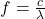(1)

f: frequency

c: speed of light in vacuum = 3*10^8 m/s

λ: wavelength

(a) For UVA the wavelength range is λ1 = 320nm to λ2 = 400nm.

The frequency range is: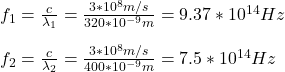frequency range: 7.5*10^14 Hz to 9.37*10^14 Hz

For UVB the wavelength range is λ1 = 280nm  to λ2 = 320nm.

The frequency range is: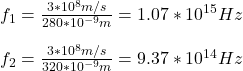frequency range: 9.37*10^14Hz to 1.07*10^15Hz

(b) The ranges for the wavenumbers are:

UVA: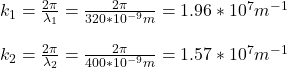wavenumber range: 1.57*10^7 m^-1 to 1.96*10^7m^-1

UVB: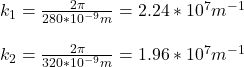wavenumber range: 1.97*10^7 m^-1 to 2.24*10^7m^-1